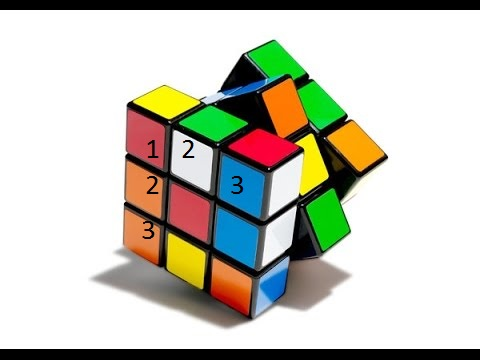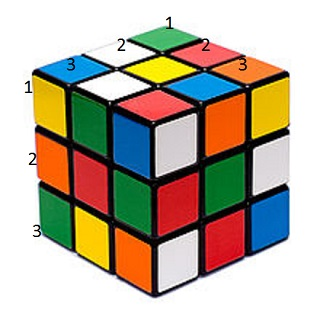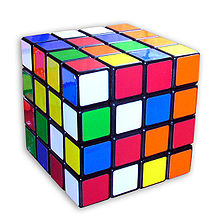# What is a Cubed Number?

Instructor: Mark Boster
Sometimes in life we need to 'cube' a number, such as when we are trying to find out how many smaller cubes are in a large cube. When we do that, we use multiplication. Read on to find out how!

## Rubik's Cube

Antonio was playing with his Rubik's Cube and having a tough time figuring it out. You may be familiar with these plastic puzzle cubes that have different colored squares on them. You have to turn it and try and get all the same colors on each side of the cube. Antonio couldn't figure it out, so he decided to try and build one himself, but he wasn't sure how many small cubes he needed to build. Let's help him!

## Find the CubesAntonio found out how many small cubes were on one layer of the Rubik's cube by counting them. He counted three across and three high. He discovered that there were 9 cubes on each layer, because he knew that 3 x 3 = 9. To find out how many cubes were in the whole puzzle, he knew that he needed to multiply not just up and down and across, but also deep, because there were multiple layers. After counting that there were 3 layers, he multiplied 3 x 3 x 3 = 27. There were 27 small cubes in his larger cube.## Cubed Numbers

Doing these kinds of equations is called 'cubing' numbers. When we cube a number, we actually multiply the number by itself three times, like this: 3 x 3 x 3. Another way to write this is 3³.

If you needed to cube the number 2, it would be written like this 2³. That means 2 x 2 x 2. Two times two is four, and four times two is eight. So 2³ = 8.

## Other Cubed NumbersTo find out how many small cubes are in a 4 x 4 x 4 cube, we would write 4³. We would start by multiplying 4 x 4 to get 16. Then we would multiply 16 x 4 again and get 64. So, four cubed is 64.

To unlock this lesson you must be a Study.com Member.

### Register to view this lesson

Are you a student or a teacher?

#### See for yourself why 30 million people use Study.com

##### Become a Study.com member and start learning now.
Back
What teachers are saying about Study.com

### Earning College Credit

Did you know… We have over 200 college courses that prepare you to earn credit by exam that is accepted by over 1,500 colleges and universities. You can test out of the first two years of college and save thousands off your degree. Anyone can earn credit-by-exam regardless of age or education level.